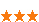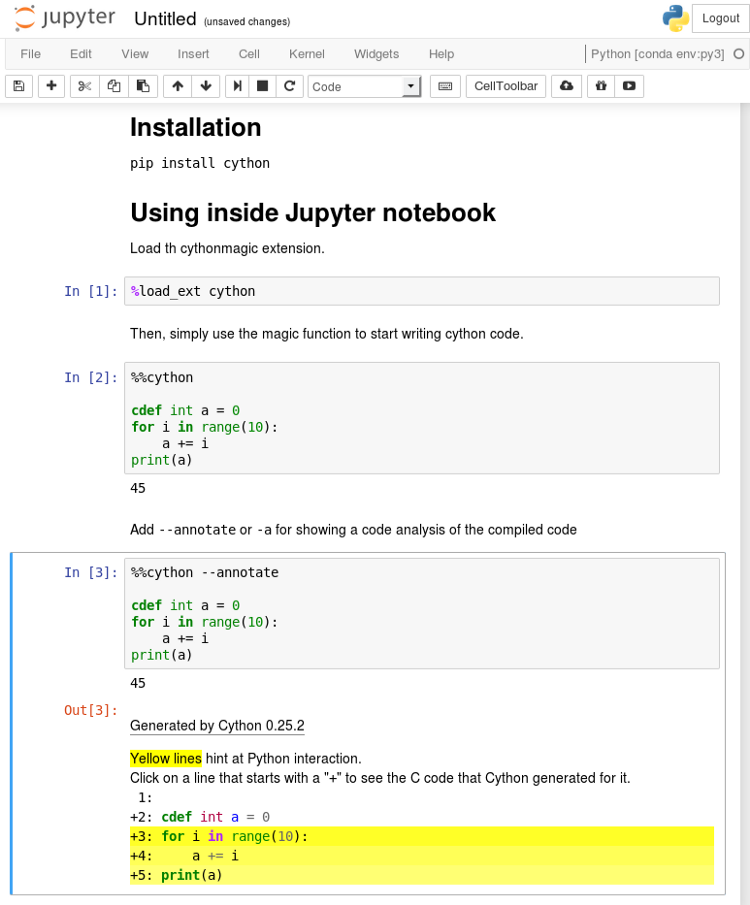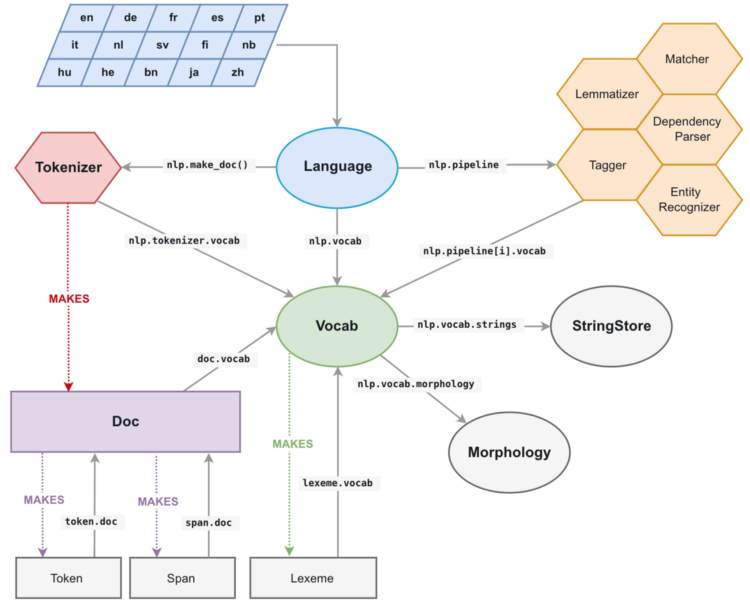您可以捐助，支持我们的公益事业。 1元 10元 50元 认证码：必填求知 文章 文库 Lib 视频 iPerson 课程 认证 咨询 工具 讲座 Modeler Code要资料订阅捐助495 次浏览     评价： 好 中 差
2019-7-24

 编辑推荐: 本文来自于outofmemory,文章主要讲解如何利用 Cython 和 spaCy 让 Python 在自然语言处理任务中的速度提高百倍。

 import cProfile import pstats import my_slow_module cProfile.run('my_slow_module.run()', 'restats') p = pstats.Stats('restats') p.sort_stats('cumulative').print_stats(30)

 from random import random class Rectangle: def __init__(self, w, h): self.w = w self.h = h def area(self): return self.w * self.h def check_rectangles(rectangles, threshold): n_out = 0 for rectangle in rectangles: if rectangle.area() > threshold: n_out += 1 return n_out def main(): n_rectangles = 10000000 rectangles = list(Rectangle(random(), random()) for i in range(n_rectangles)) n_out = check_rectangles(rectangles, threshold=0.25) print(n_out)

Cython语言是Python的超集，Python包含两种对象：

Python对象就是我们在常规Python中操作的对象，比如数字、字符串、列表、类实例・・・

Cython C对象是C或C++对象，比如双精度、整型、浮点数、结构和向量，Cython能以运行超快的低级代码编译它们。

 from cymem.cymem cimport Pool from random import random cdef struct Rectangle: float w float h cdef int check_rectangles(Rectangle* rectangles, int n_rectangles, float threshold): cdef int n_out = 0 # C arrays contain no size information => we need to give it explicitly for rectangle in rectangles[:n_rectangles]: if rectangle[i].w * rectangle[i].h > threshold: n_out += 1 return n_out def main(): cdef: int n_rectangles = 10000000 float threshold = 0.25 Pool mem = Pool() Rectangle* rectangles = mem.alloc(n_rectangles, sizeof(Rectangle)) for i in range(n_rectangles): rectangles[i].w = random() rectangles[i].h = random() n_out = check_rectangles(rectangles, n_rectangles, threshold) print(n_out)Cython代码编写为.pyx文件。这些文件被Cython编译器编译为C或C++文件，然后进一步由系统的C编译器编译为字节码文件。接着，字节码文件就能被Python解释器使用了。

 >>> import pyximport; pyximport.install() >>> import my_cython_module

Python函数是用常见关键字def定义的。它的输入和输出均为Python对象。在函数内部既可以使用Python对象，也能使用C/C++对象，同样能调用Python和Cython函数。

Cython函数是以关键字cdef定义的。可以将Python和C/C++对象作为输入和输出，也能在内部操作它们。Cython函数不能从Python环境中直接访问（Python解释器和其它纯Python模块会导入你的Cython模块），但能被其它Cython模块导入。

Cython 函数用cpdef关键字定义时和cdef定义的函数一样，但它们带有Python包装器，因此从Python环境（Python对象为输入和输出）和其它Cython模块（C/C ++或Python对象为输入）中都能调用它们。

Cdef关键字还有另一个用途，即在代码中输入Cython C/C ++。如果你没有用该关键字输入你的对象，它们会被当成Python对象（这样就会延缓访问速度）。

spaCy是我们的“护身符”。spaCy解决这个问题的方式非常智能。

StringStore对象实现了Python unicode 字符串与 64 位哈希码之间的查找映射。spaCy的内部数据结构 和spaCy相关的主要数据结构是Doc对象，它有被处理的字符串的token序列，它在C语言类型对象中的所有注释都被称为doc.c，是为TokenC结构的数组。

TokenC结构包含了我们关于每个token所需的全部信息。该信息以64位哈希码的形式保存，能够与我们刚刚看到的Unicode字符串重新关联。

 import urllib.request import spacy with urllib.request.urlopen('https:// raw.githubusercontent.com /pytorch/examples/master/word_language_model /data/wikitext-2/valid.txt') as response: text = response.read() nlp = spacy.load('en') doc_list = list(nlp(text[:800000].decode('utf8')) for i in range(10))

 def slow_loop(doc_list, word, tag): n_out = 0 for doc in doc_list: for tok in doc: if tok.lower_ == word and tok.tag_ == tag: n_out += 1 return n_out def main_nlp_slow(doc_list): n_out = slow_loop(doc_list, 'run', 'NN') print(n_out)

 import numpy # Sometime we have a fail to import numpy compilation error if we don't import numpy from cymem.cymem cimport Pool from spacy.tokens.doc cimport Doc from spacy.typedefs cimport hash_t from spacy.structs cimport TokenC cdef struct DocElement: TokenC* c int length cdef int fast_loop(DocElement* docs, int n_docs, hash_t word, hash_t tag): cdef int n_out = 0 for doc in docs[:n_docs]: for c in doc.c[:doc.length]: if c.lex.lower == word and c.tag == tag: n_out += 1 return n_out def main_nlp_fast(doc_list): cdef int i, n_out, n_docs = len(doc_list) cdef Pool mem = Pool() cdef DocElement* docs = mem.alloc(n_docs, sizeof(DocElement)) cdef Doc doc for i, doc in enumerate(doc_list): # Populate our database structure docs[i].c = doc.c docs[i].length = (doc).length word_hash = doc.vocab.strings.add('run') tag_hash = doc.vocab.strings.add('NN') n_out = fast_loop(docs, n_docs, word_hash, tag_hash) print(n_out)495 次浏览     评价： 好 中 差订阅捐助
 相关文章 手机软件测试用例设计实践 手机客户端UI测试分析 iPhone消息推送机制实现与探讨 Android手机开发（一）
 相关文档 Android_UI官方设计教程 手机开发平台介绍 android拍照及上传功能 Android讲义智能手机开发
 相关课程 Android高级移动应用程序 Android系统开发 Android应用开发 手机软件测试